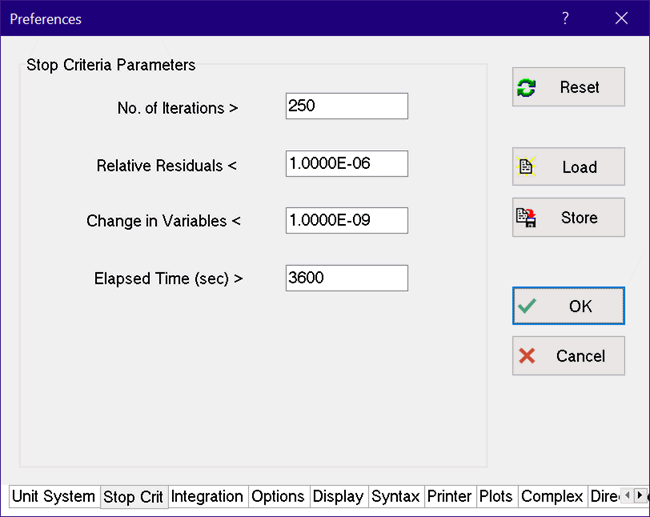Contents - Index

Stop Criteria

EES is a numerical solver and, in most problems, it uses an iterative procedure to solve the equations that you have entered. The Stop Criteria tab of the Preferences dialog, shown below, allows the user to enter parameters that control the accuracy of the iterative calculations. The calculations will be stopped if any of the first four conditions are met.The No. of Iterations must be between 1 and 32760.

Relative (as opposed to absolute) equation residuals are used to test convergence.  A relative equation residual is the difference between the left-hand and right-hand sides of an equation divided by the magnitude of the left-hand side of the equation, assuming its absolute value is greater than 1E-20.  Both the relative and absolute residuals can be viewed in the Residuals Window.  The Relative Residuals tolerance must be between 0 and 1.

EES will stop the calculations if, during an iteration, none of the variables were changed by amount greater than the value provided in the 'Change in Variables <' parameter.  To eliminate this test, enter a small value such as 1E-20 for this parameter so that the calculations are stopped by one of the other criteria.  The Change in Variables tolerance must be between 0 and 1.

The numerical algorithms used in EES attempt to solve the set of simultaneous equations to an accuracy controlled by the stopping criteria.  Because an exact solution is not obtained, it is difficult in some cases to detect a singular equation set.  Singularity can occur in several ways but the most common cause in EES is a redundant equation.  A simple example is provided by the following two equations.  These equations may appear correct, but a close examination reveals that both equations relate enthalpy to temperature at a specified pressure.  The numerical procedure used to calculate steam properties as well as the stopping criteria tolerances may prevent a direct identification of this problem.

h=enthalpy(Steam,T=T,P=100 [kPa])

T=temperature(Steam,h=h,P=100 [kPa])

If the OK button is pressed, the changes made in the Stop Criteria parameters, as well as the parameters on other tabs, will be in effect only for this session.  Note, however, that the Stop Criteria parameters are stored along with other information in the .EES or .EEZ file when the Save or Save As command is used.  The setting are restored when the file is opened with the Open command.  If the Store button is pressed, all of the information in the Preferences dialog, including the Stop Criteria, will be stored in a specified .prf file in the working directory.  Alternatively, the Load button can be used to load a previously stored .PRF file from any directory.

Note that the information in this dialog can also be set with the \$StopCriteria directive.

See also:  Integration Parameters

\$StopCriteria directive